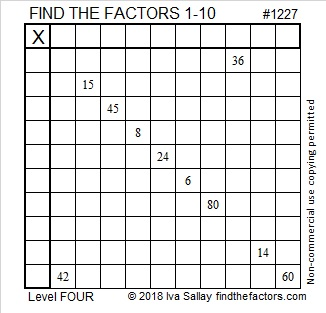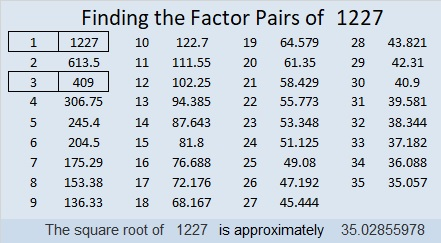# 1227 and Level 4

I’m confident you know a common factor of 42 and 60 for which ALL the factors involved are numbers from 1 to 10. That’s all you need to know to start this puzzle. Go ahead, give it a try!Print the puzzles or type the solution in this excel file: 10-factors-1221-1231

Here is some information about the number 1227:

• 1227 is a composite number.
• Prime factorization: 1227 = 3 × 409
• The exponents in the prime factorization are 1 and 1. Adding one to each and multiplying we get (1 + 1)(1 + 1) = 2 × 2 = 4. Therefore 1227 has exactly 4 factors.
• Factors of 1227: 1, 3, 409, 1227
• Factor pairs: 1227 = 1 × 1227 or 3 × 409
• 1227 has no square factors that allow its square root to be simplified. √1227 ≈ 35.028561227 is the hypotenuse of a Pythagorean triple:
360-1173-1227 which is 3 times (120-391-409)

This site uses Akismet to reduce spam. Learn how your comment data is processed.